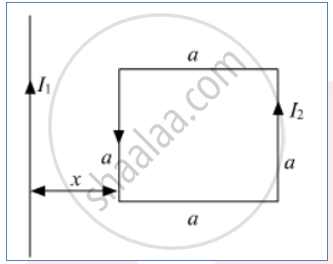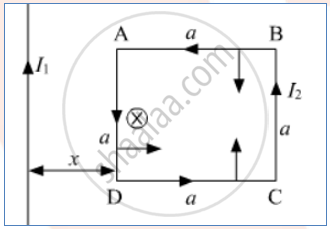# A Square Loop of Side 'A' Carrying a Current I2 is Kept at Distance X from an Infinitely Long Straight Wire Carrying a Current I1 as Shown in the Figure. Obtain the Expression for the Resultant - Physics

A square loop of side 'a' carrying a current I2 is kept at distance x from an infinitely long straight wire carrying a current I1 as shown in the figure. Obtain the expression for the resultant force acting on the loop.#### SolutionAccording to the right-hand screw rule, the magnetic field will be into the plane across the loop Force on length AD

F = Bil

F_1 = (mu_0I_1I_2a)/(2pix)

Force on length BC

F = Bil

F_2 = (mu_0I_1I_2a)/(2pi(x + a))

Force on AB and CD will be equal and opposite . Hence, they'll cancel out. Force on the loop

F_"Net" = F_1 - F_2

= (mu_0I_1I_2a)/(2pi)[1/x - 1/((x + a))]

F_("Net") = (mu_0I_1I_2a)/(2pi)[(x + a - x)/((x + a)x)] = (mu_0I_1I_2a^2)/(2pi(x + a)x)

F_("Net") = (mu_0I_1I_2a^2)/(2pix(x + a))   (Towards left)

Concept: Torque on Current Loop, Magnetic Dipole - Torque on a Rectangular Current Loop in a Uniform Magnetic Field
Is there an error in this question or solution?